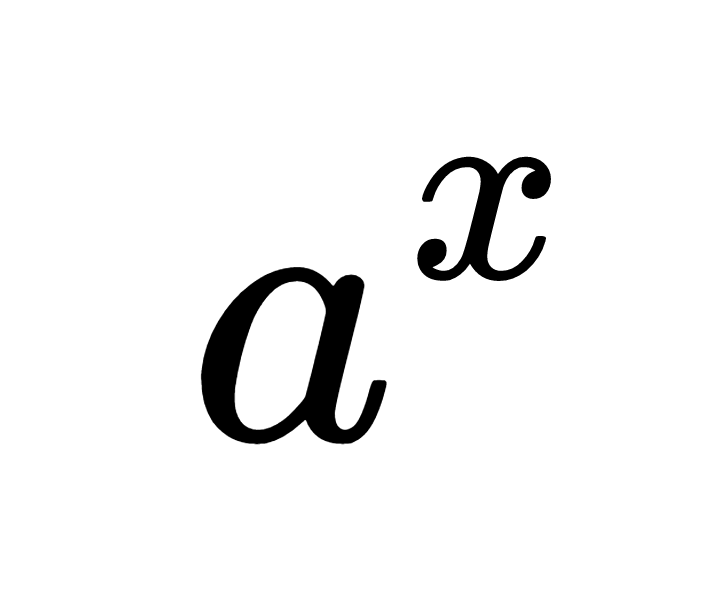# Exponent Calculator

Enter the base and exponent to calculate the result below.

ax = ?

## Results:

ax =

Learn how we calculated this below

## How to Calculate an Exponent

An exponent is the number of times the base value should be multiplied by itself. To calculate a number raised to an exponent, multiply the number by itself for each value of the exponent.

So 52 is the same as 5 × 5 and 53 is the same as 5 × 5 × 5.

Calculating an exponent is also sometimes referred to as raising a number to the nth power.

For example, calculate the result of 84.

84 = 8 × 8 × 8 × 8
84 = 4,096

Thus, 84, or 8 to the 4th power, is equal to 8 times 8 times 8 times 8, which is equal to 4,096.

## How to Calculate a Negative Exponent

What do you do if the exponent is negative? For a negative exponent rather than multiplying the number by itself the number of times for the exponent, you divide the number by itself, starting with 1.

So 5-2 is the same as 1 ÷ 5 ÷ 5 and 5-3 is the same as 1 ÷5 ÷ 5 ÷ 5.

Negative exponents are often expressed as 1 over the base with a positive exponent.

5-2 = 1 / 52

For example, calculate the result of 84.

8-4 = 1 / 84
8-4 = 1 / 8 × 8 × 8 × 8
8-4 = 1 / 4,096
8-4 = 0.000244140625

Thus, 8-4 is equal to 0.000244140625.

## Special Exponent Rules

There are some special rules with exponents that are important to remember.

When the exponent is 1, the result of raising a base to the exponent will always be equal to the base.

a1 = a

When the exponent is 0, the result of raising a base to the exponent will always be equal to 1.

a0 = 1

When the exponent is 2 you can also say that the base value is squared. When the exponent is 3 you can say that the base value is cubed.

The calculator above will handle these special cases along with negative exponents to solve the answer.

## Exponent Table

Exponent table showing the powers of 2, powers of 3, powers of 4, and powers of 5.
Powers of Two Powers of Three Powers of Four Powers of Five
20 = 1 30 = 1 40 = 1 50 = 1
21 = 2 31 = 3 41 = 4 51 = 5
22 = 4 32 = 9 42 = 16 52 = 25
23 = 8 33 = 27 43 = 64 53 = 125
24 = 16 34 = 81 44 = 256 54 = 625
25 = 32 35 = 243 45 = 1024 55 = 3125
26 = 64 36 = 729 46 = 4096 56 = 15625
27 = 128 37 = 2187 47 = 16384 57 = 78125
28 = 256 38 = 6561 48 = 65536 58 = 390625
29 = 512 39 = 19683 49 = 262144 59 = 1953125
210 = 1024 310 = 59049 410 = 1048576 510 = 9765625